# What is Multicollinearity (or Collinearlity) ?

What is (Multi)Collinearity?

To better understand the definition of collinearity, let’s start with an example.

Here we have a dataset from an ice cream store. The dataset is collected over 4 days, with each row corresponds to 1 day. We have 3 predictor variables, those are: the temperature in Celsius, the temperature in Fahrenheit and humidity; along with 1 respond variable: ice cream sales.

There is something strange about this data: we have 2 fields that contain the same information (Temperature), just on 2 different scales (Celsius and Fahrenheit).
Well, that’s called Perfect Collinearity.

When we have 2 fields and the Pearson correlation between these 2 fields is exactly 1, we say that the dataset suffers from Perfect collinearity.

When we have 3 fields and 1 of them can be formed from a linear combination of the other 2, we also call that Perfect collinearity (e.g. imagine we have 3 predictors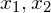and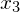such that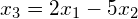).

The same goes for cases when we have n fields.

Now we know what perfect collinearity is, it is not so hard to guess the definition of high collinearity. In the case of 2 fields, high collinearity happens when the Pearson correlation of these 2 fields is high. In the case of n fields, high collinearity happens when 1 field can be approximated by a linear combination of the other (n-1) fields.

When we say a dataset is suffering from collinearity, we usually mean it has perfect collinearity or high collinearity.

The above dataset seems simple, maybe a simple model will work well on this data, sp let’s apply a Linear regressor on it. The result might be something like this: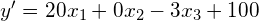where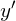is the predicted ice cream sales,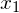is Temperature in Celsius,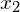is Temperature in Fahrenheit and lastly,is humidity.

This linear regression model fits ideally with our data. The error, as you can see, is 0 (try to verify it by yourself!).

Okay, above regression equation is good, but there are other equations that can produce equally goodness, for example: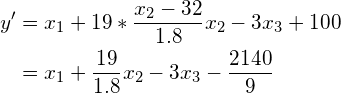You can see that the error of this model is also 0.

This gives us a big concern about evaluating the importance of predictor variables. In the first model, we have thatis very important (its weight is 20 when‘s weight is 0 and‘s weight is 3). But in the second model,is overwhelming.

We may be misled by the models. If we look at model 1, we will see that: Oh, temperature (in Fahrenheit) does not play any role in predicting ice cream sales. This is obviously false. Same goes with model 2, we may look at it and think that temperature (in Celsius) is not so important. Different models make us think differently, and it seems like both our thoughts about the importance of features are false.

So we know what is bad about collinearity. Above, I take an extreme example of perfect collinearity, but the sense also applies to high collinearity. Collinearity makes our predictive model volatile, which, in turn, misleads us of the feature importance.

Note that, surprisingly, collinearity does NOT affect the performance of most models. As we can see in the above example, the error rates of both models are 0. A rare exception when multicollinearity worsens a model is in the case of Naive Bayes models, which require predictors to be independent of each other.

How to avoid collinearity?

• Eliminate high-correlation columns. We can iterate through all columns and check the Person correlation amongst them. High (or perhaps very high) correlated columns should be taken care of. A drawback of this approach is that we can only test the correlation of each pair of columns, we can not detect the case when one column is correlated with a linear combination of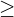2 other columns (e.g.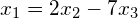). The good news is that those cases are much less likely to happen as the cases when 2 predictor variables are highly correlated to each other.
• Use dimension reduction techniques. PCA is often the choice. The bad thing about this approach is that when we do dimension reduction, we also lose the ability to interpret the data.
• Use Variance Inflation Factor (VIF). This is a technique that was specifically created for dealing with multicollinearity. Unlike using correlation – you can only see the relation of 1 column versus another, VIF can help us detecting multicollinearity in a 1-versus-all manner. What VIF does is basically to compute, for each predictor, how precisely it is predicted using a linear combination of the other predictors. That is, we regress a predictor using all other predictors, and get the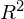. The higher the, the higher the level of multicollinearity this predictor has.

Summary

(Multi)Collinearity is the correlation of 1 predictor variable with a linear combination of1 other predictor variables.

We can fix the problem by eliminating high-correlation columns, using dimension reduction techniques, VIF, etc. However, be noted that each method comes with its own drawbacks.

Questions:

1. I only care about the performance of my predictive model, should I pay attention to collinearity?

2. Perfect collinearity, high collinearity, low collinearity and no collinearity, which of them are completely good?

3. When a column can be formed by a non-linear combination of other columns (e.g.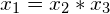), is this called collinearity and is it bad?